# CHSE Odisha Class 12 Math Solutions Chapter 7 Continuity and Differentiability Ex 7(e)

Odisha State Board Elements of Mathematics Class 12 CHSE Odisha Solutions Chapter 7 Continuity and Differentiability Ex 7(e) Textbook Exercise Questions and Answers.

## CHSE Odisha Class 12 Math Solutions Chapter 7 Continuity and Differentiability Exercise 7(e)

Differentiate the following functions by proper substitution.
Question 1.
sin-1 2x$$\sqrt{1-x^2}$$
Solution:
y = sin-1 2x$$\sqrt{1-x^2}$$   [Put x = sin θ
= sin-1 (2 sin θ . cos θ)
= sin-1 sin 2θ = 2θ = 2 sin-1 x.
$$\frac{d y}{d x}$$ = $$\frac{2}{\sqrt{1-x^2}}$$

Question 2.
tan-1 $$\frac{2 x}{1-x^2}$$
Solution:
y = tan-1 $$\frac{2 x}{1-x^2}$$
= tan-1 $$\frac{2 \tan \theta}{1-\tan ^2 \theta}$$ = tan-1 (tan 2θ)
= 2θ = 2 tan-1 x
∴ $$\frac{d y}{d x}$$ = $$\frac{2 x}{1-x^2}$$Question 3.
tan-1 $$\sqrt{\frac{1-t}{1+t}}$$
Solution: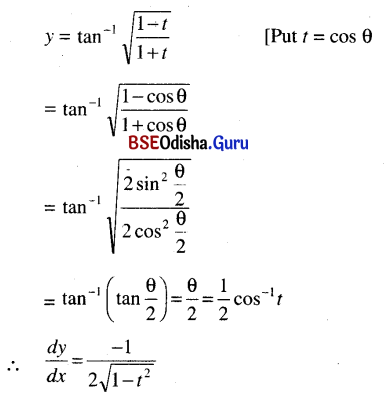Question 4.
$$\left[\left(\frac{1+t^2}{1-t^2}\right)^2-1\right]^{\frac{1}{2}}$$
Solution: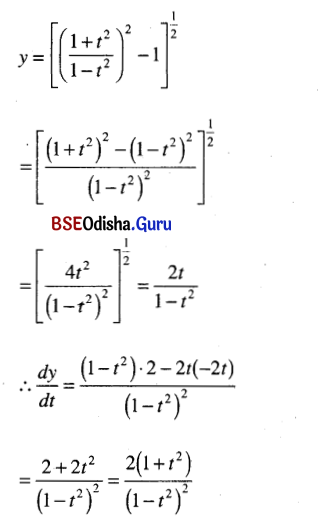Question 5.
tan-1 $$\left(\frac{\sqrt{x}+\sqrt{a}}{1-\sqrt{x a}}\right)$$
Solution: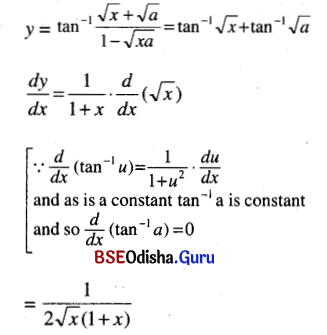Question 6.
sin-1 ($$\frac{2 x}{1+x^2}$$)
Solution:
y = sin-1 $$\frac{2 x}{1+x^2}$$  [Put x = tan θ
= sin-1 $$\frac{2 \tan \theta}{1+\tan ^2 \theta}$$ = sin-1 sin θ
= 2θ = 2 tan-1 x
∴ $$\frac{d y}{d x}$$ = $$\frac{2 x}{1+x^2}$$

Question 7.
sec-1 $$\left(\frac{\sqrt{a^2+x^2}}{a}\right)$$
Solution: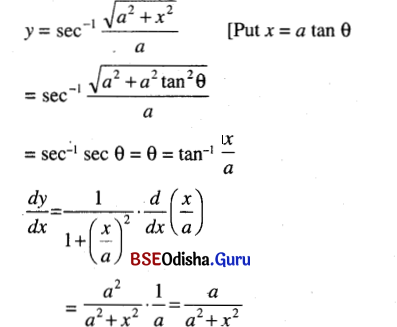Question 8.
sin-1 $$\left(\frac{2 \sqrt{t^2-1}}{t^2}\right)$$
Solution: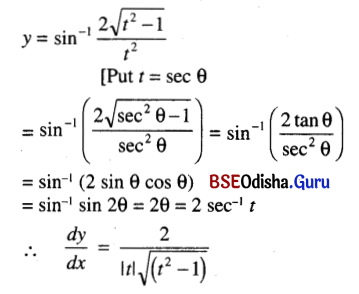Question 9.
cos-1 $$\left(\frac{1-t^2}{1+t^2}\right)$$
Solution:
y = cos-1 $$\left(\frac{1-t^2}{1+t^2}\right)$$ [Put t = tan θ
= cos-1 $$\frac{1-\tan ^2 \theta}{1+\tan ^2 \theta}$$
= cos-1 cos 2θ = 2 tan-1 t
∴ $$\frac{d y}{d x}$$ = $$\frac{2}{1+t^2}$$

Question 10.
cos-1 (2t2 – 1)
Solution:
y = cos-1 (2t2 – 1) [Put t = tan θ
= cos-1 (2 cos2 θ – 1)
= cos-1 cos 2θ = 2θ = 2 cos-1 t
∴ $$\frac{d y}{d x}$$ = – $$\frac{2}{\sqrt{1-t^2}}$$